# Calculus 3 : Dot Product

## Example Questions

1 2 10 11 12 13 14 15 16 18 Next →

### Example Question #171 : Dot Product

Find the dot product of the vectors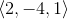and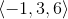Explanation:

To find the dot product of two vectors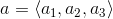and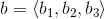, we apply the formula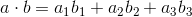Using the vectors from the problem statement, we get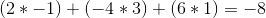### Example Question #172 : Dot Product

Find the dot product of the vectors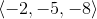and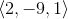Explanation:

To find the dot product of two vectorsand, we apply the formulaUsing the vectors from the problem statement, we get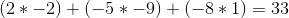### Example Question #173 : Dot Product

Find the dot product of the vectors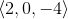and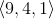Explanation:

To find the dot product of two vectorsand, we use the formulaUsing the vectors from the problem statement, we get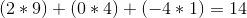### Example Question #174 : Dot Product

Find the dot product of the vectors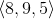and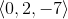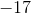Explanation:

To find the dot product of two vectorsand, we use the formulaUsing the vectors from the problem statement, we get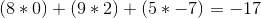### Example Question #175 : Dot Product

Solve: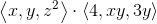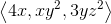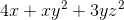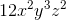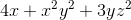Explanation:

The dot product of two vectors is equal to the sum of the product of the corresponding components (for example,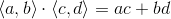)### Example Question #176 : Dot Product

Find the dot product of the vectors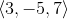and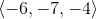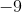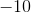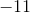Explanation:

To find the dot product of two vectorsand, you use the following formulaUsing the vectors from the problem statement, we get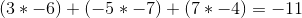### Example Question #177 : Dot Product

Find the dot product of the vectors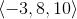and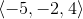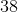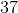Explanation:

To find the dot product of two vectorsand, you use the following formulaUsing the vectors from the problem statement, we get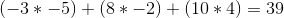### Example Question #178 : Dot Product

Find the dot product of the vectors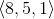and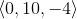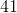Explanation:

To find the dot product between two vectorsand, you use the formula:Using the vectors from the problem statement, we get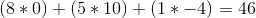### Example Question #179 : Dot Product

Find the dot product of the vectorsand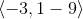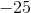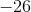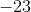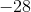Explanation:

To find the dot product between two vectorsand, you use the formula:Using the vectors from the problem statement, we get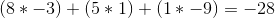1 2 10 11 12 13 14 15 16 18 Next →